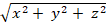Chapter 16.7, Problem 4E

Chapter
Section
Textbook Problem

Suppose that f(x, y, z) = g(), where g is a function of one variable such that g(2) = −5. Evaluate ∬S f(x, y, z) dS, where S is the sphere x2 + y2 + z2 = 4.

To determine

To find: The value of Sf(x,y,z)dS .

Explanation

Given data:

S be the sphere x2+y2+z2=4 ,

f(x,y,z)=g(x2+y2+z2) and g(2)=5 .

The total and surface area of a sphere (SdS) is 4πr2 .

Find Sf(x,y,z)dS .

Sf(x,y,z)dSSg(x2+y2+z2)dSSg(2)dS

Substitute –5 for g(2) ,

Sf(x,y,z)

Still sussing out bartleby?

Check out a sample textbook solution.

See a sample solution

The Solution to Your Study Problems

Bartleby provides explanations to thousands of textbook problems written by our experts, many with advanced degrees!

Get Started

263

Applied Calculus for the Managerial, Life, and Social Sciences: A Brief Approach

Evaluate the integrals in Problems 1-26.

Mathematical Applications for the Management, Life, and Social Sciences

Differentiate. y=x2+1

Single Variable Calculus: Early Transcendentals

Finding a Limit In Exercises 11-28, find the limit. limx6x2

Calculus: Early Transcendental Functions (MindTap Course List)

The equation of the tangent plane to for is:

Study Guide for Stewart's Multivariable Calculus, 8th

Find f(1)+f(2)+f(3)+....+f(20), given that f(x)=3x4.

Finite Mathematics for the Managerial, Life, and Social Sciences